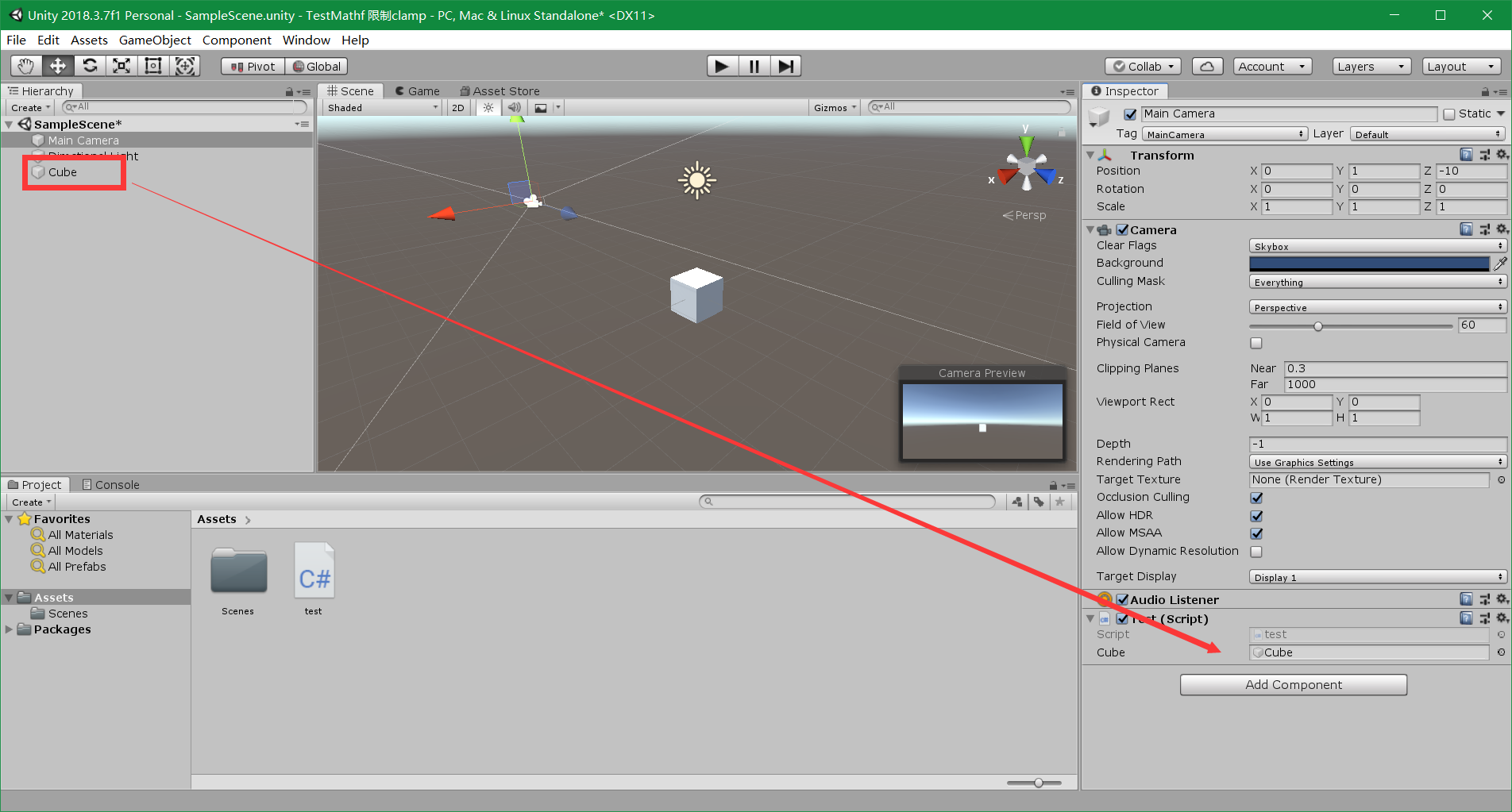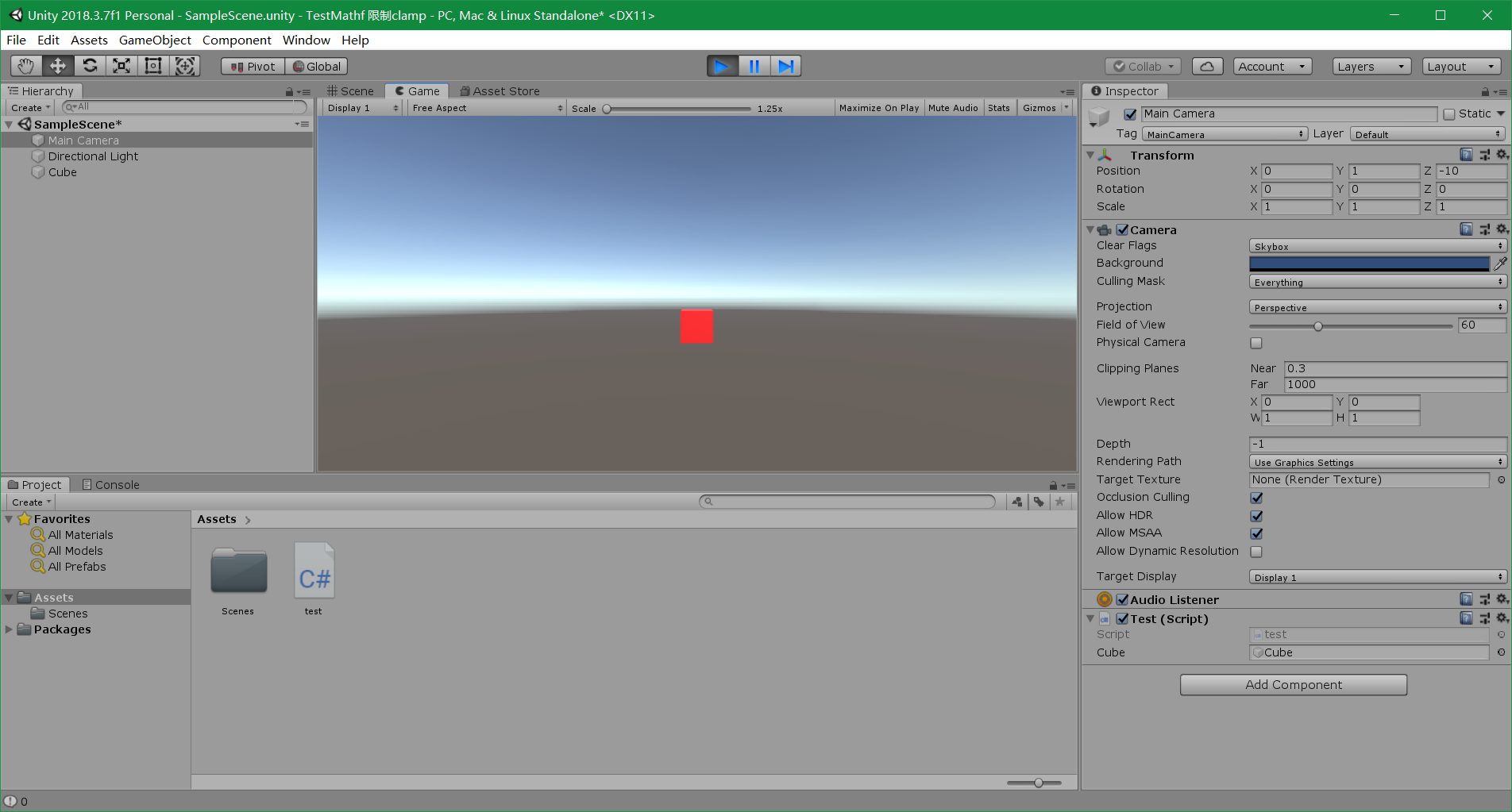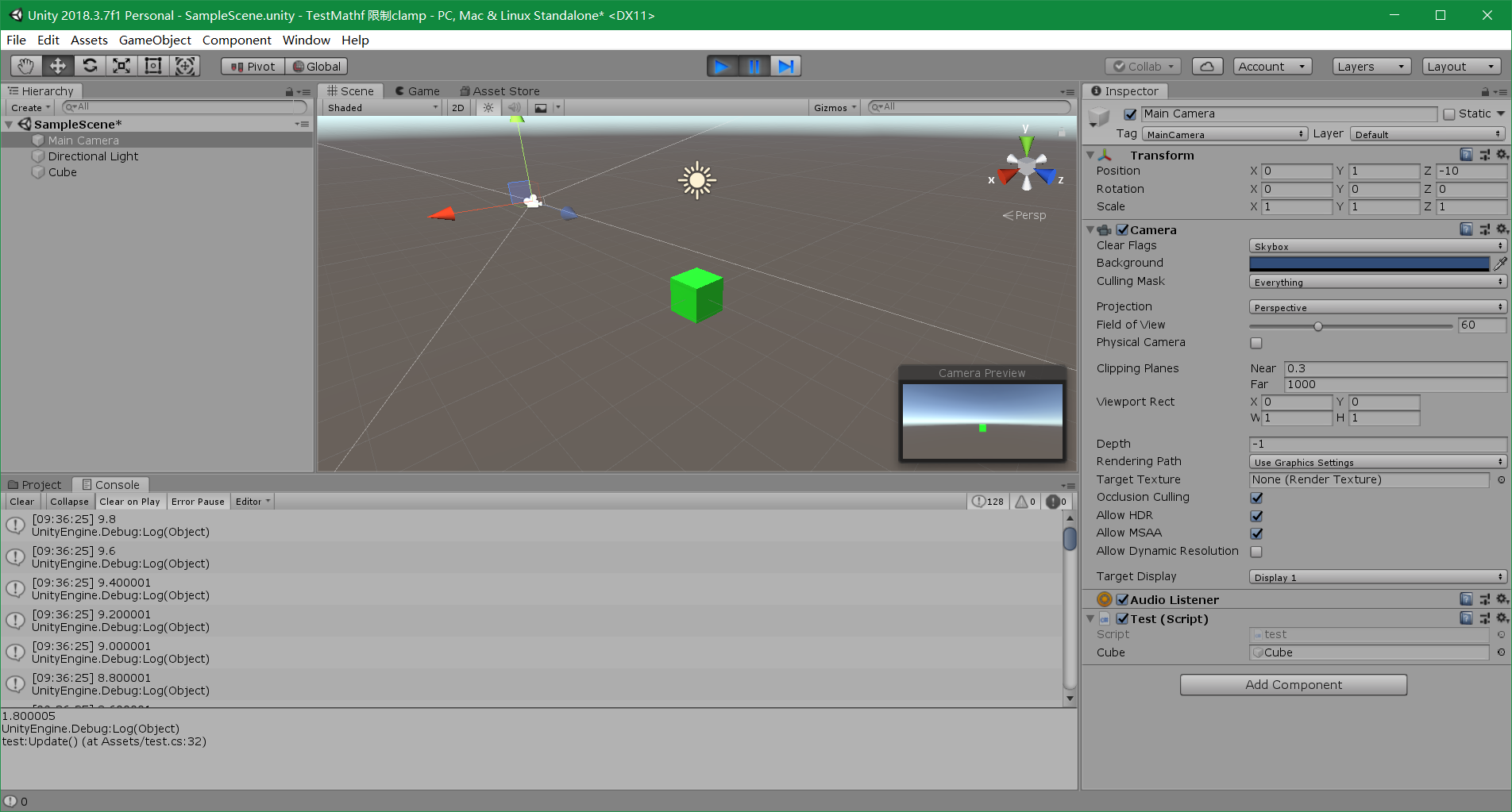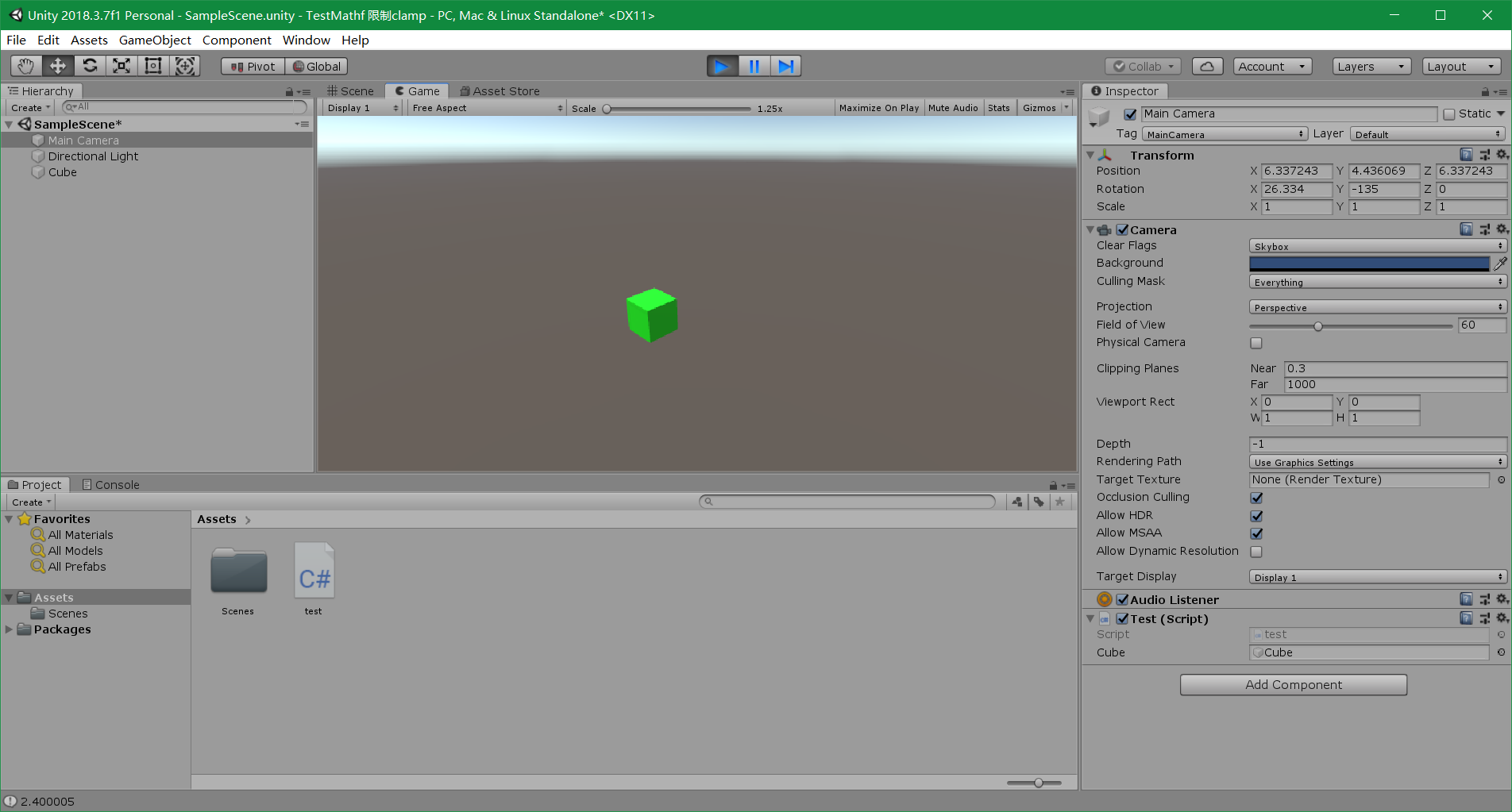# 1. Mathf’s Clamp static method

## 1.1. public static float Clamp(float value, float min, float max);

Parameters

value The floating point value to restrict inside the range defined by the min and max values.
min The minimum floating point value to compare against.
max The maximum floating point value to compare against.

Returns
float The float result between the min and max values.

Description
Clamps the given value between the given minimum float and maximum float values. Returns the given value if it is within the min and max range.

## 1.2. public static int Clamp(int value, int min, int max);

Parameters

value The integer point value to restrict inside the min-to-max range
min TThe minimum integer point value to compare against.
max The maximum integer point value to compare against.

Returns
float The int result between the min and max values.
Description
Clamps the given value between a range defined by the given minimum integer and maximum integer values. Returns the given value if it is within min and max.

Returns the min value if the given value is less than the min value. Returns the max value if the given value is greater than the max value. The min and max parameters are inclusive. For example, Clamp(10, 0, 5) will return a maximum argument of 5 and not 4.

## 1.3. Test

### 1.3.1. Foreground

Create a 3D object Cube and a test C# script, then bind the 3D object Cube to a variable that named cube in the test C# script.### 1.3.2. Code

using System.Collections;
using System.Collections.Generic;
using UnityEngine;

public class test : MonoBehaviour
{
public GameObject cube;
float a = 10;
// Start is called before the first frame update
void Start()
{

}

// Update is called once per frame
void Update()
{
float x = Input.GetAxis("Horizontal");//-1~1

//When we press A or D button, the cube will turn red;
if (Mathf.Abs(x)>0)
{
cube.GetComponent<Renderer>().material.color = Color.red;
}
else
{
cube.GetComponent<Renderer>().material.color = Color.green;
}

//The value of a goes from 9.8 to 0.
a = Mathf.Clamp(a - 0.2f, 0, Mathf.Infinity);
Debug.Log(a);

//Cube will move as the value of a changes.
//cube.transform.Translate(new Vector3(a, 0, 0) * Time.deltaTime);
cube.transform.position = new Vector3(a, 0, 0);
}
}



### 1.3.3. Result

• When we press A or D button, the cube will turn red;• The value of a goes from 9.8 to 0.• Cube will move as the value of a changes.# 2. Mathf’s Lerp static method

## 2.1. public static float Lerp(float a, float b, float t);

Parameters

a The start value.
b The end value.
t The interpolation value between the two floats.

Returns
float The interpolated float result between the two float values.

Description

• Linearly interpolates between a and b by t.
• The parameter t is clamped to the range [0, 1].
• When t = 0 return a.
When t = 1 return b.
When t = 0.5 return the midpoint of a and b.

Example of usage

• For Mathf.Lerp(1, 2, t)：
When t ≤ 0 return 1.
When t ≥ 1 return 2.
When t = 0.5 return the midpoint of a and b.
When 0<t<0.5, returned value closer to 1.
When 0.5<t<1, returned value closer to 2.

The code to do this is shown below.

using System.Collections;
using System.Collections.Generic;
using UnityEngine;

public class test : MonoBehaviour
{
float a = 0, t = -1;
// Start is called before the first frame update
void Start()
{

}

// Update is called once per frame
void Update()
{
/*
For Mathf.Lerp(1, 2, t)：
When t ≤ 0 return 1.
When t ≥ 1 return 2.
When t = 0.5 return the midpoint of a and b.
When 0<t<0.5, returned value closer to 1.
When 0.5<t<1, returned value closer to 2.
*/
Debug.Log("" + Mathf.Lerp(1, 2, t) + ", " + t);
t += 0.01f;
}
}



# 3. Homework

Sin Returns the sine of angle f.
Sqrt Returns square root of f.
Pow Returns f raised to power p.
using System.Collections;
using System.Collections.Generic;
using UnityEngine;

public class NewBehaviourScript : MonoBehaviour
{
// Start is called before the first frame update
void Start()
{
print("Mathf.Sin(10)=" + Mathf.Sin(10));
print("Mathf.Sqrt(9)=" + Mathf.Sqrt(9));
print("Mathf.Pow(10, 2)=" + Mathf.Pow(10, 2));
}

// Update is called once per frame
void Update()
{

}
}01-161万+

#### unity Mathf 数学运算(C#)

03-13614

#### Unity3D之Vector3.Dot和Vector3.Cross的使用

02-21187

#### Unity Gameobject类测试

03-2174

#### Unity Vector3 class

05-231825

#### 【Unity】Mathf的常用函数Abs，Ceil，Floor，Lerp等

02-246940

#### Unity3D中Mathf.SmoothDamp函数使用与Mathf 数学运算(C#)

10-21267

#### Unity中Mathf函数

05-2382

#### Unity中Mathf移动的五种方法

04-04515

#### unity之Mathf常用函数

07-072390

#### Unity3d Mathf数学运算说明

05-264万+

#### Unity3D中的线性插值Lerp()函数解析

03-26413

#### 最新微信小程序源码

04-1460万+

#### 微信小程序源码-合集1

03-1315万+

#### 程序员请照顾好自己，周末病魔差点一套带走我。

02-1918万+

#### Java基础知识面试题（2020最新版）

03-0114万+

#### 技术大佬：我去，你写的 switch 语句也太老土了吧

03-0414万+

#### 和黑客斗争的 6 天！

03-064092

#### Intellij IDEA 实用插件安利

03-087万+

#### 女程序员，为什么比男程序员少？？？

04-257万+

#### 总结了 150 余个神奇网站，你不来瞅瞅吗？

03-1013万+

#### 副业收入是我做程序媛的3倍，工作外的B面人生是怎样的？

03-1019万+

#### MySQL数据库面试题（2020最新版）

03-1211万+

#### 如果你是老板，你会不会踢了这样的员工？

03-1312万+

#### 我入职阿里后，才知道原来简历这么写

03-163253

#### 魂迁光刻，梦绕芯片，中芯国际终获ASML大型光刻机

03-181万+

#### 优雅的替换if-else语句

03-199万+

#### 离职半年了，老东家又发 offer，回不回？

03-194万+

#### 2020阿里全球数学大赛：3万名高手、4道题、2天2夜未交卷©️2019 CSDN 皮肤主题: 编程工作室 设计师: CSDN官方博客点击重新获取扫码支付